## When do I take which distribution?

### Contents

1.  Distributions for the occurrence of the risk

1.1.  Bernoulli distribution

1.2.  Binomial distribution

1.3.  Poisson distribution

2.  Distributions for the effects of risk

2.1.  Constant distribution

2.2.  The uniform distribution

2.3.  Triangular, PERT and modified PERT distribution

2.4.  Trapezoidal distribution

2.5.  Custom Distributions

2.6.  Normal distribution

2.7.  Lognormal distribution

2.8.  Weibull distribution

2.9.  Automatic calibration of the distributions

2.10.  Compound distribution

3.  Application of distributions in ERM

One of the most frequently asked questions in enterprise risk management is, 'when do I use which distribution to represent enterprise risk?' It is asked by beginners as well as by experienced risk managers who are faced with the task of opening the chapter of quantitative risk management for the first time in the company.

There is no canonical answer to this question. It is not the case that the financial damage of a loss of production due to flooding of the plant area generally obeys a triangular or a normal distribution. The same applies to all other types of risk.

However, we can consider the properties of many important distributions and then consider which distribution with their properties fits best in a specific situation.

We can also discuss how to determine the parameters of a distribution and whether this is practically possible in the given situation. Because only if we can parameterize a chosen distribution, we can use it.

In fact, this is a central and delicate step in the survey and assessment of risks in the risk management process, because many risk experts who have to assess the factual context are generally not risk managers, but are responsible for completely different tasks in the company. Nevertheless, they must be in a position to comment on these issues.

In the everyday life of companies, the determination of parameters therefore often plays the central role in the selection of distributions in Enterprise Risk Management (ERM). Practically only distributions that are easy to explain and can be parameterized with the help of expert assessments are used. Parameterizations through the statistical evaluation of data on risk play only a secondary role.

We will therefore concentrate on this group of distributions for the time being. It includes above all the constant distribution, the triangular distribution and the PERT distribution, but also the uniform distribution and the trapezoidal distribution. Many companies use them to model all risks. Less well known is the modified PERT distribution, which has a shape parameter that can be used to adjust the flattening of the long side, and custom distributions, where the user can draw the density himself.

All of these distributions are bounded on both sides. Distributions unbounded on one or both sides are less often parameterized with expert estimates. Where they are nevertheless used, the normal and lognormal distributions are usually employed. Sometimes also the Weibull distribution. All of these are classic textbook distributions.

Thus the status quo is guided by a very pragmatic rule. 'Use distributions you know and can parameterize.' In what follows, we will portray these distributions, discuss their uses, and show how they can be parameterized using expert judgments and algorithms.

In Enterprise Risk Management, the modeling of risks is divided into two steps. First, the occurrence of risks is presented, and in the second step, the impact of the risk in the event that it occurred.

We take this dichotomy as an outline and group the representation into distributions that model the entry side of a risk and distributions that are used to describe the potential loss amounts.

## 1.Distributions for the occurrence of the risk

To top

Distributions that model the entry side of a risk count how often the risk actually materializes in a period.

In principle, all distributions that take the counting numbers 0, 1, 2, 3, etc. as values can be used for this purpose. In practice, however, three distributions are discussed and two of them are actually used. These are the Bernoulli, Poisson and binomial distributions, with the first two being used in practice.

### 1.1.Bernoulli distribution

To top

The Bernoulli distribution is the classic occurrence model in enterprise risk management. Its position dates back to the time when a risk was represented by a probability of occurrence and an impact - two numbers each. The use case was very specifically described operational risks in a one-period view.

The Bernoulli distribution describes a generalized coin toss. There are exactly two states. An event ("head" or, transferred to our case, "risk materializes") occurs or does not occur. Multiple occurrences are not possible.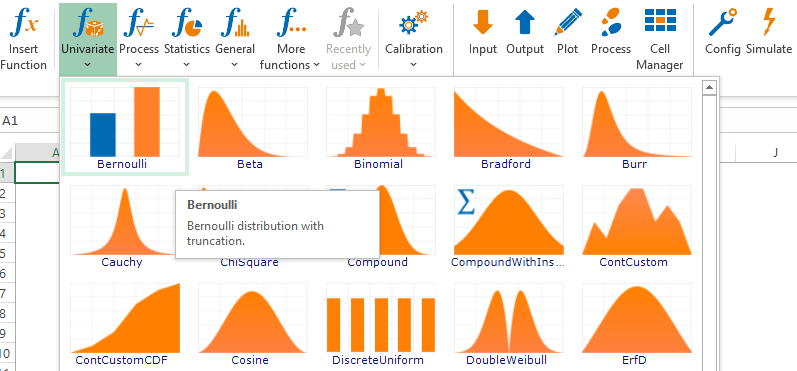Figure 1- The Bernoulli Distribution on the Risk Kit Toolbar

The distribution has one parameter, the probability of occurrence P of the risk under consideration.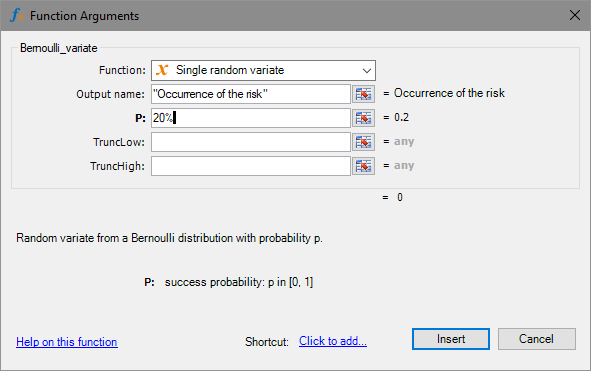Figure 2- Input Dialog of the Bernoulli Distribution in Risk Kit

In ERM, the probability of occurrence (OP) is in most cases determined by expert assessment. This is also necessary because in ERM many risks are included in the analysis that have not yet occurred in everyday life but are considered possible.

If risk experience is available in the company, the OP can also be estimated by dividing the number of observed occurrences of the risk by the number of observation years. Thus, if the risk has occurred for example twice in the last 10 years, according to this logic the OP would be estimated at 20%.

In order to broaden the scope of risk knowledge, it is quite possible to include experience from the market. If a risk has occurred at other companies, there may be much to suggest that it could also happen at your own company.

The evaluation of data for determining the probability of occurrence is all the more reliable the more representative occurrences there are for the risk. The rarer the risk, the more difficult and error-prone it becomes. If, in the extreme case, the risk has never occurred, it is not possible to reach the goal without expert assessments.

In the simulation, the Bernoulli distribution takes the values 0 and 1. The 1 represents the occurrence of the risk.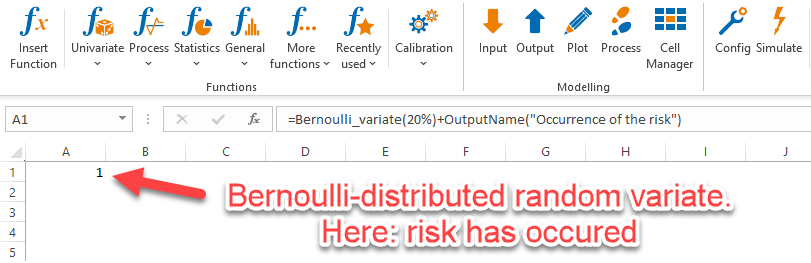Figure 3- Bernoulli distributed random number

This property of the Bernoulli distribution, that a risk either cannot occur or can occur exactly once, but never more than once, is an essential criterion for its application.

In many ERM models that look at the evolution of risks over multiple periods, the Bernoulli distribution creates an inconsistency in the model. In multi-period models, risk occurrence is simulated in each period. If a Bernoulli distributed risk has occurred in period 1 in a simulation run, this risk is henceforth blocked for the period and cannot occur any further. However, as soon as the next fiscal year (period 2) has begun, the risk is unlocked again and can occur again from January.

The same contradiction arises when periods are divided. If one changes from an annual to a quarterly view in a Bernoulli model, the risk can suddenly occur four times as often over the original period of one year as before. Conversely, if one switches to a 5-year view for strategic risks, for example, the risk will occur less frequently, even if the probabilities of occurrence are correctly adjusted to the changed periods.

The Bernoulli distribution is best suited as a model for the occurrence of a risk if this risk can only occur once, regardless of the delimitation of the periods.

For the original use case 'specific operational risks with a one period observation horizon' this criterion is very well fulfilled. A specific product is taken off the market only once. A named bridge collapses only once, etc.

This changes in the case of more generally formulated descriptions of operational risks ('One of our products has to be taken off the market', 'A supply route becomes impassable and the supply chain is interrupted'). Analogously for extended risk terms.

An extension of the modelling of risk entries therefore allows multiple entries of a risk in a period. The two most important tools for this are the binomial and Poisson distributions.

Mathematically, the Bernoulli distribution is the building block from which these two (and many other) distributions are constructed. It is therefore a direct generalization.

### 1.2.Binomial distribution

To top

A binomial distribution arises when we have a fixed number n of Bernoulli experiments in which only 0 or 1 (success or failure, risk fails or risk occurs) can come out.

The total number of successes on n trials takes a value in the count numbers 0, 1, 2, ..., n.

Example: If I operate a wind farm of 8 wind turbines and cannot easily replace one turbine, 0 to 8 wind turbines may fail at the same time.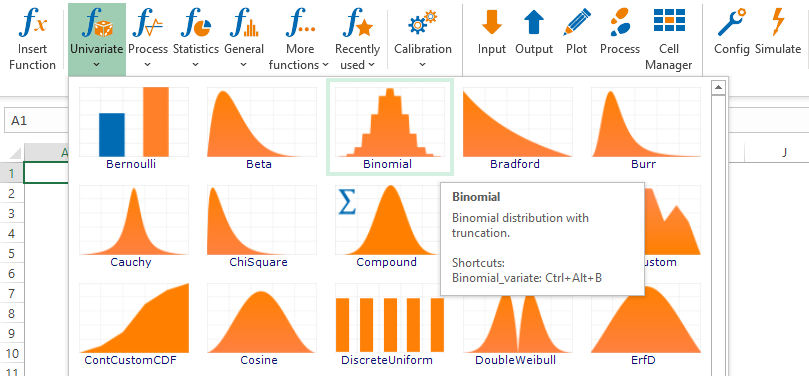Figure 4- The Binomial Distribution on the Risk Kit Toolbar

The binomial distribution has two parameters. The number of trials n and the probability of success in each trial p.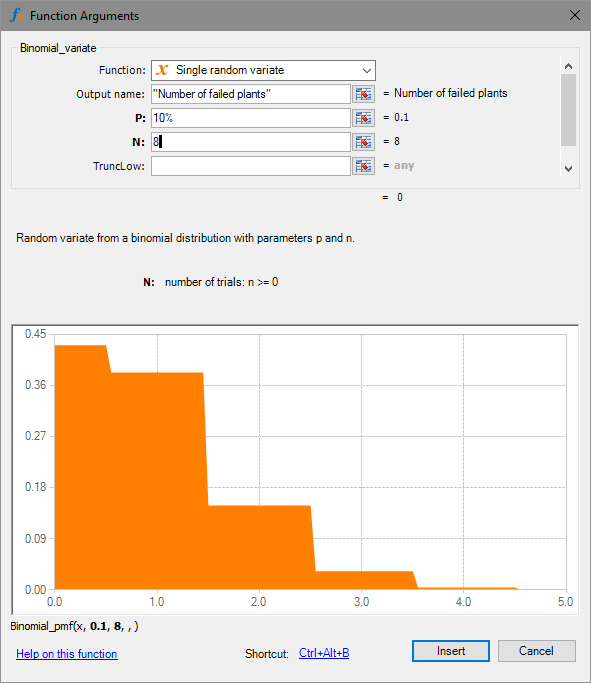Figure 5- Input Dialog of the Binomial Distribution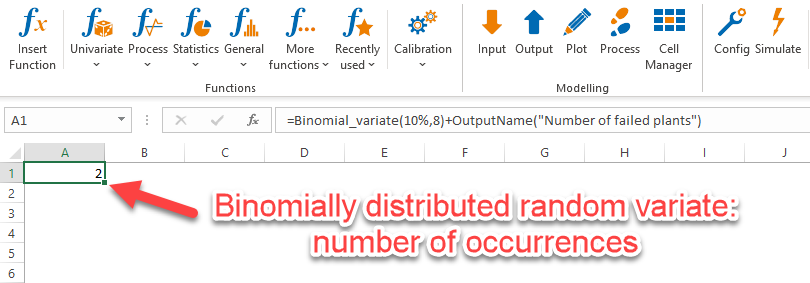Figure 6- Binomial distributed random number

An important assumption of the binomial distribution is that the probability of success remains the same for each experiment. In practice, this can be true, but it can also be a limitation.

For the wind farm in the example, the assumption of equal probabilities of occurrence for the risk of failure of a wind turbine would be well met if the turbines were very similar in model, load and age. However, if the wind farm is a mixture of smaller and larger turbines of different types and with different operating durations, it might be more realistic to consider a separate risk for each turbine.

The binomial distribution as a model for the number of system failures would also not be ideally suited if a system only failed temporarily and went back into production after repair. In this case, it would be possible for individual wheels to fail several times and for more failures to occur in individual cases than there are plants in the park.

In a multi-period model, if the binomial distribution is used, there may be dependence between periods if the maximum frequency of occurrence in a period is changed by the number of claims in a previous period.

If, in the example, two wind turbines have permanently failed in period 1, only 6 of the original turbines will remain intact in the subsequent periods, so that the value of n would have to be reset here. Additional damage would further reduce the number of still intact turbines.

By breaking down the risk into one risk per plant, which is finally dismantled after failure, this case can also be simplified in the same way as above, so that the time dependencies arise by themselves.

Due to these complexities and the general possibility of splitting the risk into risks with Bernoulli distributed occurrences, the binomial distribution is very rarely used for modelling occurrence frequencies of risks in ERM.

In ERM, due to the enterprise-wide use of the model and the involvement of a large number of people, a certain standardization of the model components is generally desired.

In technical models of large-scale plants it is different. Here, detailed representations of smaller, homogeneous groups of plants, as described in the example of the wind farm, are a standard element in which the binomial distribution has a central place.

### 1.3.Poisson distribution

To top

If an event occurs over a period of time with constant probability ('intensity') and these occurrences are independent of each other, its frequency of occurrence is Poisson distributed.

It takes values on the count numbers 0, 1, 2, .....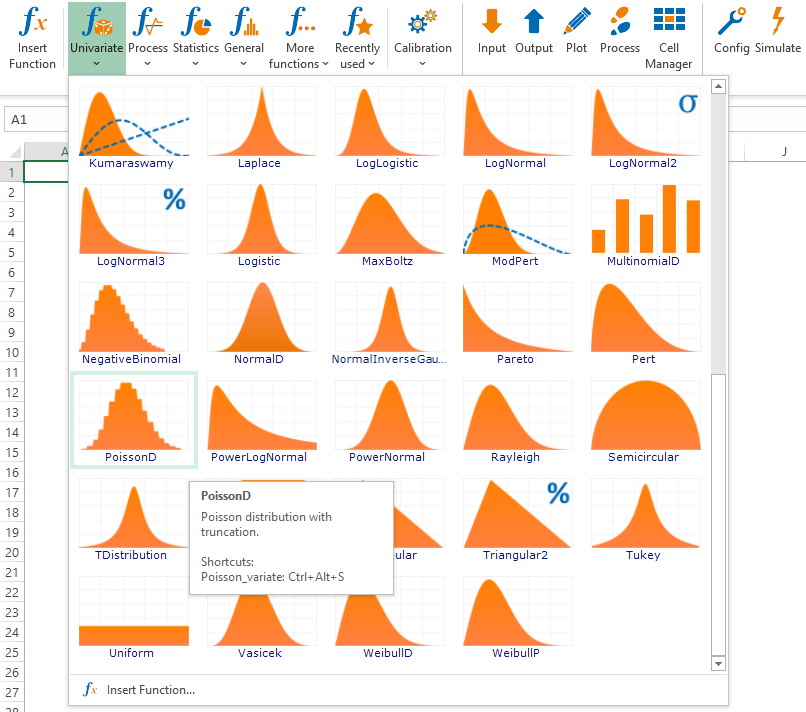Figure 7- The Poisson Distribution on the Risk Kit Toolbar

Its parameterization is done via the expected frequency of occurrence lambda of the risk. This is a major advantage of the distribution in the context of the ERM process, as the expected frequency of occurrence is clearly understandable to experts and can therefore be determined with good justification. It can also be obtained very well from data as an expected value of the frequencies of occurrence, if such data are available.

Example: In the wind farm example, the number of lulls, i.e. periods of low wind of a certain minimum duration, is an important factor for the quality of the site and the profitability of the plant.

The expected number of doldrums per year can be determined from the weather data of the past years.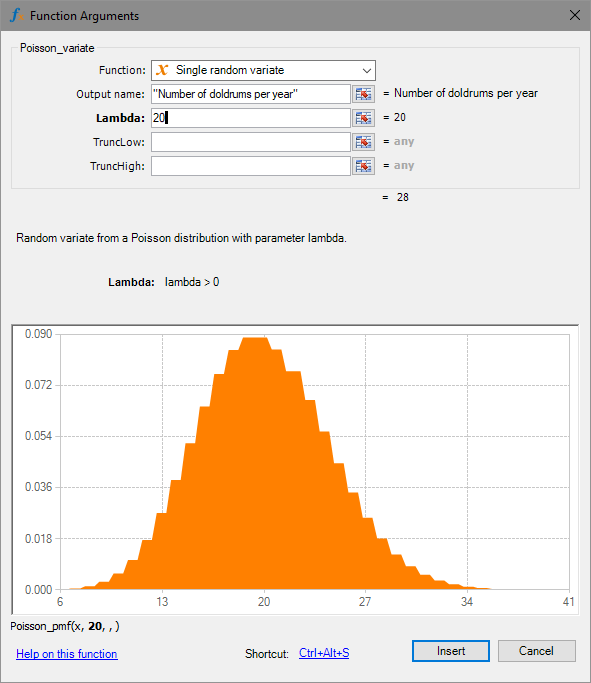Figure 8- Input Dialog of the Poisson Distribution

In this example, an important property of the Poisson distribution is that we do not need to specify a maximum number of doldrums.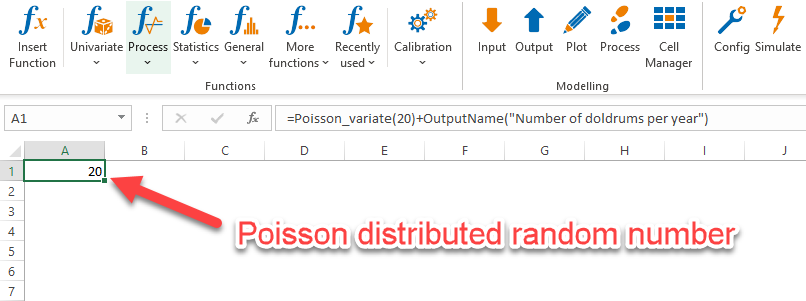Figure 9- Poisson distributed random number

Poisson and binomial distributions are approximately interchangeable in many cases. This is always the case when the expected occurrence frequency lambda = n * p is small compared to n. The deviations between the two distributions are then usually so small that they play no practical role in the ERM process. Both distributions even become equal when, for a given expected occurrence frequency, n becomes large.

Figure 10gives an example of the comparison of both distributions for lambda = 1.5, p = 15% and n = 10.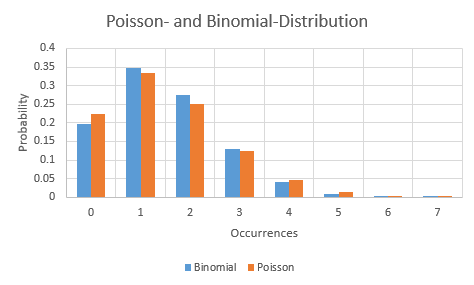Figure 10 - Poisson and binomial distribution in comparison

Because of these properties, the Poisson distribution is the most widely used model in ERM for representing frequencies.

## 2.Distributions for the effects of risk

To top

In order to fully assess a risk, after the description of the occurrence of the risk, the amount of loss after occurrence is relevant. It is generally assumed that each occurrence causes an individual loss. Thus, if a risk occurs more than once, the total loss is the sum of the individual losses. We will discuss this in detail in the section on the compound distribution, which evaluates a random number of losses of a random amount in each case.

The distribution most commonly used in companies in the past for single loss occurrence is the constant distribution. This point-based representation is often perceived as unrealistic. Attempts are therefore being made to replace it with ranges. These ranges may well include opportunities. Distributions that are often used for this purpose are the uniform, triangular, PERT and trapezoidal distributions.

All of these distributions are directly related to the classic business best- and worst-case consideration and were originally used for estimating operational risks, i.e. operational losses. In these cases, a worst case in the sense of 'tear down and rebuild' is often well defined.

The situation is different for risks whose effects cannot be so easily capped. What is the worst-case scenario in the event of a pandemic? Some companies have failed precisely at this point with a realistic assessment of the risks. After all, they have included the pandemic in the risk inventory (which most of the companies concerned have not done), but have underestimated the impact by a factor of tens. The assessment is quickly rendered moot by such an error.

Distributions often used for this application are the lognormal and the Weibull distribution. Both are extreme value distributions and can therefore potentially also assume very large values with a small probability.

### 2.1.Constant distribution

To top

Risk management textbooks of the past have described a risk by a probability of occurrence and an impact. The impact is a fixed number. This 'constant distribution' is still the way things are in many companies today.

The representation of a risk by two values has advantages.

• A risk has a short and precise looking profile.

• All risks are standardised. Very different types of risk can be reported so well.

• Risk quantification seems straightforward.

• Both variables ('OP and loss amount') can be compared graphically. If necessary, categorised and underlaid with coloured gradations, this results in the risk map.

The constant distribution is so simple in its technical implementation that you don't need any tools for it. It provides random numbers that you know in advance, so you don't have to draw them at all. It can be represented in Excel by a simple number.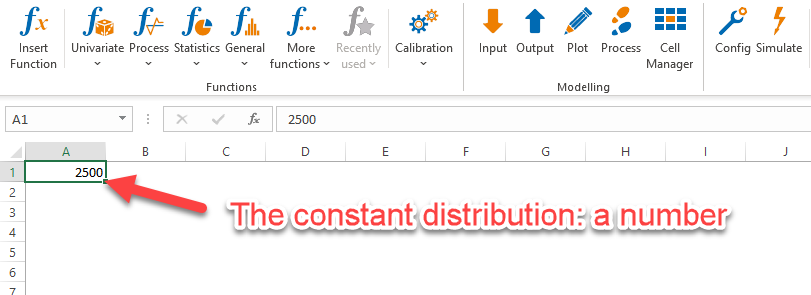Figure 11- The Constant Distribution in Excel

On closer inspection, however, describing the impact of a risk in terms of a fixed figure often turns out to be a well-meaning illusion. Only in the case of a few risks is the exact amount of the loss known in advance when it occurs. A threatened contractual penalty or the certain replacement of a wear part could be such cases.

In most contexts, however, the impact of a risk can realistically only be defined in terms of bandwidths, often a very wide range.

### 2.2.The uniform distribution

To top

If one follows the paradigm of a range for the possible losses after the occurrence of risk, the uniform distribution corresponds to this picture in a natural way.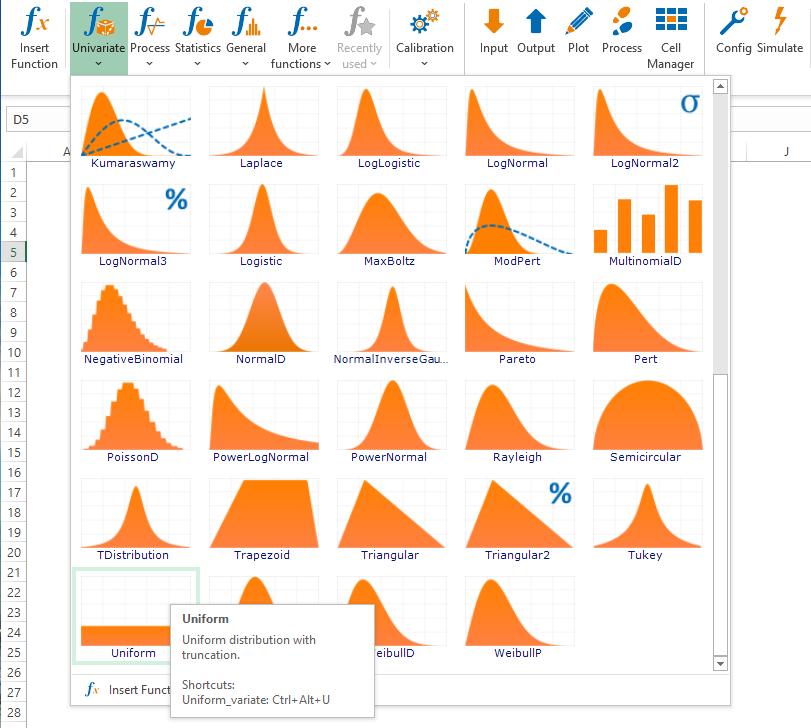Figure 12- The Equal Distribution on the Risk Kit Toolbar

It takes values between a minimum A and a maximum B andits density has exactly the shape of a band.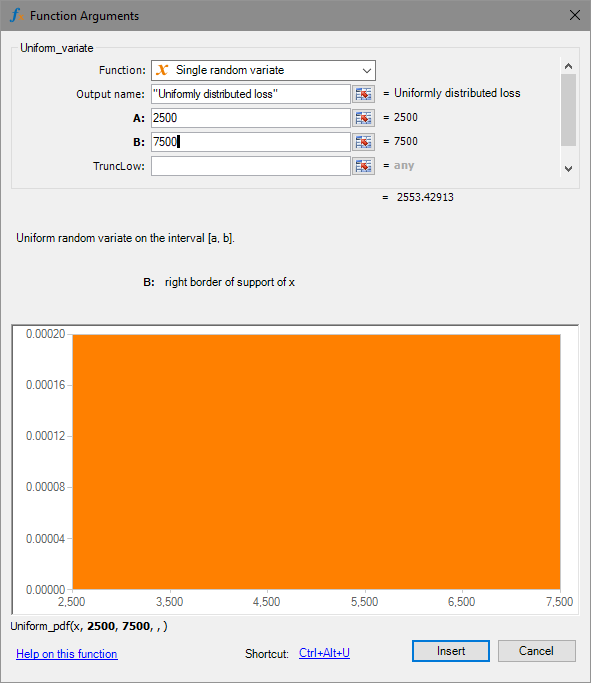Figure 13- Input Dialog of the Uniform Distribution

Each value between A and B isassigned the same probability.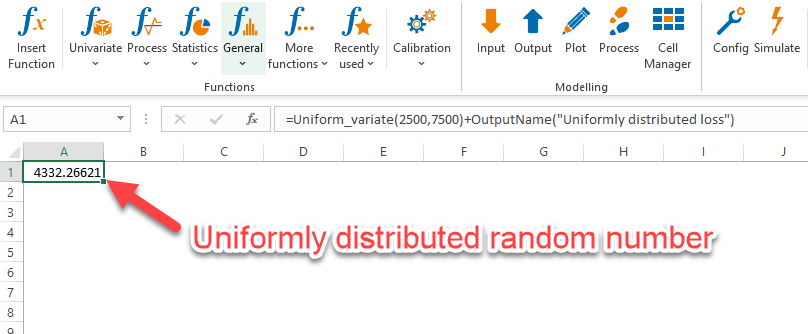Figure 14- Equally Distributed Random Number

Uniformly distributed random events occur in many games, often in their integer version. The sides of a die show uniformly distributed on top. Playing cards are shuffled in a uniformly distributed manner. The ball of a roulette wheel selects a digit in a uniformly distributed manner. The uniform distribution here is just the epitome of fairness.

We can harness this property in ERM.

In the wind farm example, if we had data on the length of doldrums in recent years, we can number the observed records and draw a number across the uniform distribution. The cost of the simulated doldrum is then the length of the doldrum drawn times the lost revenue per unit time.

With this approach, we avoid the need to determine the distribution of the lull lengths and can directly access the observed data. However, we will also never simulate a lull that is longer than the longest observation in our sample.

Other approximately uniformly distributed quantities include the exact location where a pipeline will leak, a rope will break, or a cable will snap.

Although it captures the bandwidth picture so directly, the uniform distribution in the ERM is often not immediately plausible. One important reason for this is that the density does not evolve steadily. Damage outside A and B have density 0. Values in this range are not assumed. Exactly at A, however, the density then jumps to a high level, remains there until B is reached, and then falls abruptly back to 0.

It would be more understandable to have a model in which the unadopted range merges seamlessly into the range in which the damages occur. This will give rise to the introduction of further distributions in a moment.

Precisely this disadvantage of the uniform distribution, namely that damages in the extreme ends A and B ofthe value range are assumed with an unrealistically high probability, is paradoxically a reason why the uniform distribution is often used, at least transitionally.

On the one hand, the choice of distribution may signal that the exact shape of the frequency trend in the damage area is either not known or has not been investigated. Something like a most likely value or the more precise shape of the distribution are then not known. Here, therefore, an approximate solution is made clear (Pierre-Simon Laplace's indifference principle).

On the other hand, the uniform distribution fluctuates more strongly on the range of values than the alternatives triangle, PERT or trapezoid. The overall risk therefore becomes more sensitive to this risk factor than if another model is chosen. Therefore, if a risk does not have a large effect on the outcome when the uniform distribution is chosen, this will not change much even when the effects are more refined. The choice of extreme values A and B isthen initially decisive. If they are correct, a more differentiated representation of the impact distribution may not be worthwhile and the above-mentioned indifference principle can justifiably be applied.

### 2.3.Triangular, PERT and modified PERT distribution

To top

The triangular and PERT distributions are an important alternative to the uniform distribution when designing the range of possible losses from a risk. Here, the loss experience is structured over three points, the minimum, the mode and the maximum.

The mode is the high point of the density of the distribution. It is also referred to as the 'most likely value' because it is the most likely to observe damage in an environment around this point.

The determination of these key data by means of the questions 'In which range do the losses lie minimally?', 'In which range do the losses lie maximally?' and 'In which range do we most likely expect to see losses?' is very easily possible even for risk experts who do not normally deal with the parameterisation of probability distributions in everyday life. This suggestive power is so strong that many companies base their entire ERM on these two loss distributions alone.